Updating search results...

# 12 Results

View
Selected filters: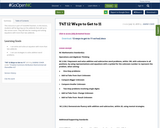Conditional Remix & Share Permitted
CC BY-NC-SA
Rating
0.0 stars

This resource is part of Tools4NCTeachers.

In this lesson, students will be figuring out the addends that add up to the correct sums. They will also be creating and solving equations with more than two addends.

Subject:
Mathematics
Material Type:
Assessment
Lesson
11/06/2019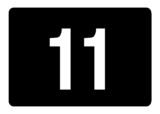Unrestricted Use
CC BY
Rating
0.0 stars

This resource is from Tools4NCTeachers.&nbsp;&nbsp;In this lesson, students will be figuring out the addends that add up to the correct sums.&nbsp; They will also be creating and solving equations with more than two addends.Extension and differentiation ideas are provided.

Subject:
Mathematics
Material Type:
Activity/Lab
Lesson
Lesson Plan
Author:
DAWNE COKER
06/23/2020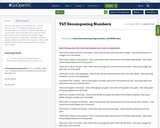Conditional Remix & Share Permitted
CC BY-NC-SA
Rating
0.0 stars

This resource is part of Tools4NCTeachers.

This file contains a set of 17 problem solving tasks around decomposing numbers within 10 (where both addends are unknown). Each of the tasks has its own recording sheet, which may be copied for the students. Page 1 contains a general scoring rubric, and page 2 contains sample student work.

We'd love to see your student work samples! Please consider remixing this task to include work samples.

Subject:
Mathematics
Material Type:
Assessment
Formative Assessment
11/06/2019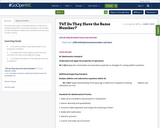Conditional Remix & Share Permitted
CC BY-NC-SA
Rating
0.0 stars

This resource is part of Tools4NCTeachers.

In this lesson, students explore the communitive property in the context of a word problem to determine if students have the same number of items

Subject:
Mathematics
Material Type:
Lesson
Lesson Plan
11/06/2019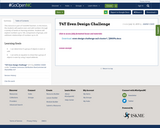Conditional Remix & Share Permitted
CC BY-NC-SA
Rating
0.0 stars

This resource is part of Tools4NCTeachers.

In this lesson, the students will explore even and odd numbers through a variety of hands-on learning activities. Students will explore numbers up to 100, comparisons of groups, and odd/even relationships of numbers up to 20.

Subject:
Mathematics
Material Type:
Lesson
11/06/2019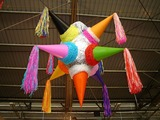Unrestricted Use
CC BY
Rating
0.0 stars

This resource is from Tools4NCTeachers.In this lesson, students will solve higher-level word problems involving three addends.&nbsp; The rich problems allow for multiple solutions, so the focus will be on student thinking rather than correct answers.&nbsp; Students will explore various strategies to solve and will be asked to attempt new strategies, including writing matching equations, once that has been modeled by others.&nbsp; &nbsp;This resource includes a lesson plan, answer key, and printable exit ticket.&nbsp; Remix this lesson to include extension activities of photos of student work samples.

Subject:
Mathematics
Material Type:
Lesson
Lesson Plan
Author:
DAWNE COKER
06/14/2020Unrestricted Use
CC BY
Rating
0.0 stars

This resource is from Tools4NCTeachers.&nbsp;&nbsp;This is a set of five&nbsp;(5)&nbsp;tasks, which may be&nbsp;used for instruction or assessment.&nbsp; Each file contains a separate task,&nbsp;rubric, and recording sheets.&nbsp; The word problems in these tasks focus&nbsp;around put-together/take apart problems where both addends are unknown.Remix this resource to include extension ideas or addtional tasks.

Subject:
Mathematics
Material Type:
Activity/Lab
Assessment
Formative Assessment
Author:
DAWNE COKER
06/10/2020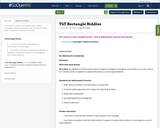Conditional Remix & Share Permitted
CC BY-NC-SA
Rating
0.0 stars

In this lesson, students use square tiles to create rectangles using the vocabulary rows and columns. They write an equation to represent the total.

Subject:
Mathematics
Material Type:
Lesson
Lesson Plan
11/06/2019Unrestricted Use
CC BY
Rating
0.0 stars

This resource is from Tools4NCTeachers.In this lesson, students use their understanding of word problems to sort problems based on type.&nbsp; The focus for students in this lesson shifts from solving word problems to analyzing word problem structures in order to group like problems.&nbsp; The purpose is to help students understand and visualize problems so they will know how to write equations that match and know what operation is needed to solve.&nbsp; This lesson focuses on sorting addition and subtraction word problems with a specific focus on Take Apart and Put Together types.&nbsp; The lesson, however, can be adjusted to use with any problem types you notice students need more experience with. &nbsp;You could also adjust the number size in the problems to use this lesson at different times in the year.&nbsp;

Subject:
Mathematics
Material Type:
Lesson
Lesson Plan
Author:
DAWNE COKER
06/14/2020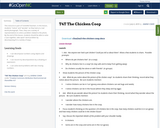Conditional Remix & Share Permitted
CC BY-NC-SA
Rating
0.0 stars

This resource is part of Tools4NCTeachers.

In this lesson, students "notice" and "wonder" as they look at a real world photograph. Then, they use a variety of representations to solve a problem related to the photo.

By the end of this lesson, students should be able to solve a
"put together, take apart" word problem by decomposing 8 in a variety of ways.

Subject:
Mathematics
Material Type:
Lesson
Lesson Plan
11/06/2019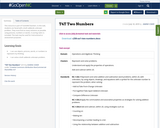Conditional Remix & Share Permitted
CC BY-NC-SA
Rating
0.0 stars

This resource is part of Tools4NCTeachers.

In this task, students are faced with a both addends unknown problem. They must find as many solutions as possible using pictures, numbers or words. A scoring rubric is included. The task may be used for instructional or assessment purposes.

Subject:
Mathematics
Material Type:
Assessment
Formative Assessment
11/06/2019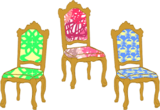Unrestricted Use
CC BY
Rating
0.0 stars

This resource is from Tools4NCTeachers.In this lesson, students explore the commutative and associative properties of addition as they solve word problems with multiple solutions.&nbsp; This lesson will help students learn to represent and solve problems with equations that have three addends. This file contains answer keys, student recording pages, and extension problems.

Subject:
Mathematics
Material Type:
Lesson
Lesson Plan
Author:
DAWNE COKER